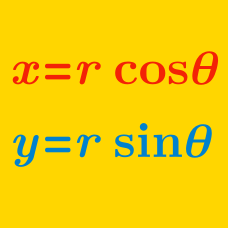Algebra

# Polar Coordinates: Level 4 Challenges

How many complex numbers $a + bi$ are there where $a$ and $b$ are integers and

$|a + bi| \leq 5 ?$

Details and assumptions:
$|a+bi|$ denotes the modulus or absolute value.

$\large\begin{cases} {56x +33y = -\frac y{x^2+y^2} } \\ {33x -56y = \frac x{x^2+y^2} } \end{cases}$

Given that $x,y$ are complex numbers that satisfy the system of equations above and that $|x| + |y|$ equals $\frac pq$ for coprime positive integers $p,q$, evaluate $6p-q$.

Let $z_1 = 6+i$ and $z_2 = 4-3i$.
Let $z$ be a complex number such that $\text{arg}\left(\dfrac{z-z_1}{z_2-z}\right)=\dfrac{\pi}{2}$, and $|z-(5-i)|$=$\sqrt{m}$.

Find $m.$


Details and Assumptions:

• $\text{arg}(x)$ is the argument of $x$.
• $z_1,z_2,$ and $z$ are complex numbers.

Let $x$ be a solution to the equation $x^2+x+2014=0$.

Find the value of $\lim\limits_{n\to \infty} \sqrt[\Large n]{\prod_{i=1}^{2n}\left|\dfrac{x^i}{2014^{\frac{i-1}{2}}}+1\right|}.$

Let $z_{1}$ and $z_{2}$ be the two complex roots of the equation $z^{2} +az+ b =0$, where $a$ and $b$ are real numbers. Further, assume that the origin, $z_{1}$ and $z_{2}$ form an equilateral triangle. Then:

×